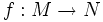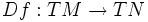# Tangent bundle

This article defines a basic construct that makes sense on any differential manifold
View a complete list of basic constructs on differential manifolds

## Definition

### Loose definition

The tangent bundle of a differential manifold$M$, denoted$TM$, is defined as a differential manifold with a bundle map to$M$ such that the fibre at every point is identified canonically with the tangent space at that point.

## Functoriality

Further information: Tangent bundle functor The tangent bundle to a differential manifold is associated to it functorially. Thus, if$f:M \to N$ is a smooth map between differential manifolds, there is an induced map$Df:TM \to TN$.

## Facts

### Sections

A section of this bundle is termed a: vector field
The sheaf of sections is termed the: sheaf of derivations of a manifold

A section of a general vector bundle over a differential manifold is an association, to every point on the differential manifold, of a vector in the fibre vector space above it. Thus, a section of the tangent bundle associates, to every point, a tangent vector to it. Sections of the tangent bundle are thus the same as vector fields.

### Dual bundle

The dual bundle to this vector bundle is termed the: cotangent bundle

### Tensor powers of the tangent bundle

Tensor powers of the tangent bundle are also important for various purposes, and sections of these tensor powers are termed tensor fields.

Further, sections of the$k^{th}$ tensor power of the cotangent bundle are differential$k$-forms.

### Tensors of mixed type

We are often interested in the vector bundles obtained by taking a tensor power with the tangent bundle repeated$p$ times and the cotangent bundle repeated$q$ times. Sections of such a vector bundle are termed tensors of type$(p,q)$.